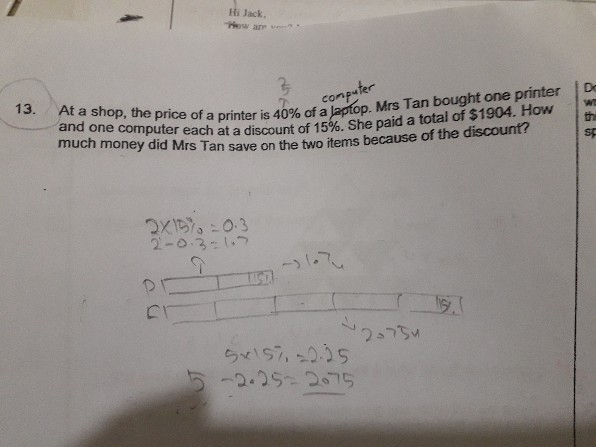QuestionHow to solve

Price of computer = 10U, So price of printer = 4U

Total price = 10u + 4u = 14u.

14u =1904, 1u = 1904/14  = 136\$

Computer = 10u = 1360\$

printer = 4u = 4 x 136 = 544\$

Computer

Discounted price = 1360\$, Original price = 1600\$, Saving = 240\$

Printer

Discounted price = 544\$, original price = 640\$, Saving = 96\$

Total savings = 240 + 96 = 336\$

0 Replies 0 Likes